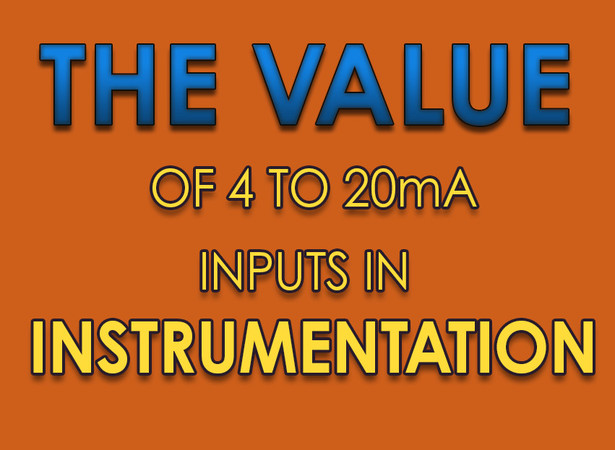# THE VALUE OF 4-20mA INPUTS

Data acquisition systems typically provided support for one or more of the following input types…. Strain gages and strain gage based transducers, high and low level DC inputs, LVDTs, thermocouples,   digital I/O, RTDs, pressure, and accelerometers to name a few.  In recent years, there has been an increase in the usage of 4 to 20 milliamp (mA) based transducers to measure many of the inputs listed above as an alternative to standard voltage driven inputs.

Starting with the commonly known Ohm’s Law, which states the V = IR, we can represent this as the simple circuit shown below: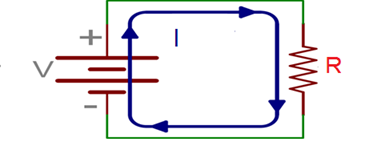One reason is that 4-20mA inputs conform to an industry standard, ISA 50.00.01-1975 (R2012) which assures the users of this type input of a common connectivity process as well as  a clear representation of zero signal (4mA) and maximum signal(20 mA). That is, when a 4 to 20mA does not perform as expected due to an open circuit, miswired input or simply no current flow, it produces an output of zero milliamps (0 mA), removing all doubt as to whether or not the input is behaving properly.  This is something that is not so clear with voltage base inputs, where 0 volts could represent a valid output of the sensor.  But it could equally represent of non-function input, thus making the interpretation of “true zero” more difficult.  Another reason for usage of this type input is that when running connections over long distances, there is little or no drop in output as normally seen with common voltage based type inputs.  In fact, increasing or decreasing the supply voltage does not alter the output of a 4-20mA input since most inputs contain a transmitter that regulates the current.  This keeps the current running through the loop “constant”.  Additionally, if the cabling connected to a 4-20mA input are insignificantly affected by electromagnetic fields like the voltage-based counterparts.  Lastly, if cost is an issue, this type of sensor only uses two conductors/cables to complete the circuit.

There are some shortcomings related using 4 to 20mA type sensors.  Only one sensor type can be used with a single current loop.  So for high channel counts this may not be ideal.  If multiple sensors are needed, then the multiple current circuits could produce ground loops and/or cross talk.  For the sake of this discussion, we’ll focus on using a single 4 to 20mA sensor with a data acquisition system. As mentioned earlier, these systems can support one or more types of inputs such as strain gages, high level DC inputs, or similar.  Micro-Measurements produces various data acquisition, most of which provide connectivity for strain gages and high level inputs to name a few.  Connecting a 4 to 20mA input to other of these inputs requires some care are each has limitations as to the amount of voltage that is allow for each channel type.

Strain gage inputs channels are typically limited to about 80 mV of input for the sensor bridge.  This inputs is often described as a “low level” input.  As for “high level” inputs, they usually represent values greater than 80 mV.  Frequency they are described as accepting voltages up to +/-10 volts.  In these two cases, a simple circuit can be used to show how to connect a 4 to 20mA input to each.  What’s required to successfully do this is to use of a shunt resistor across the existing current loop at S+ and S-, which is produced by connecting the data acquisition system to the power source and then the power source to the 4 to 20mA input as shown below.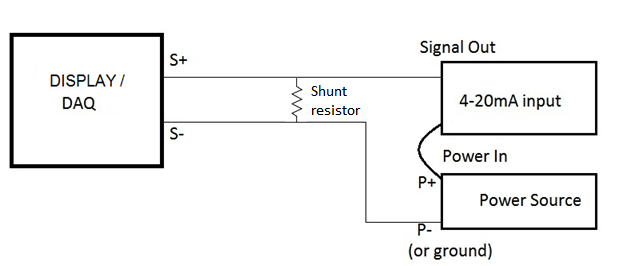So, what is the “RIGHT” value for the shunt resistor to assure that we do not cause harm to system by accidently overloading the input?  The answer goes back to Ohm’s law which states that our Voltage(V) is proportional to the product of our Current (I) and our Resistance (R).  So, using the fact that we are connecting to a 4 to 20mA input, we simply need to determine what voltage we desire to have at our DAQ.  For the strain gage based input, let’s suppose that our desired input is 4mV at 4mA and 20mV at 20mA.  Using Ohm’s Law (V=IR), the shunt resistor that would work for us would be a 1 Ohm resistor as shown below: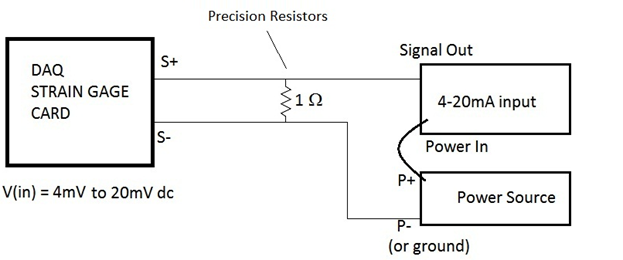However, for a high level input, there’s much more voltage that is allowable, so let’s suppose that we wanted to see an output range of 1 to 5 Vdc that would be proportional to 4 and 20 mA respectively.  Then, in this case, the proper shunt resistor that would produce the desired output would be 250 Ohm resistor as shown below: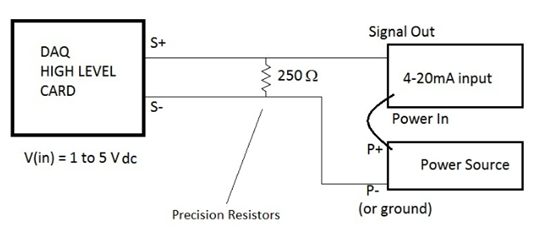For stability in such circuits, VPG bulk metal foil resistors are ideal for the shunt resistors in applications (http://www.vishaypg.com/docs/11041/stype.pdf) where process control base monitoring is critical.Donielle Dockery

Field Design Engineer